Reconstruction of the 2019 Long Wood formation 1.Draw a circle. Draw and extend the horizontal and vertical centerlines. 2.Construct the inscribed equilateral triangle of circle 1, pointing up. 3.Copy circle 1 three times, to the angular points of triangle 2. 4.Construct the circumscribed hexagon (regular 6-sided polygon) of circle 1, pointing to the right. 5.Construct a circle with the upper side of hexagon 4 as its centerline. 6.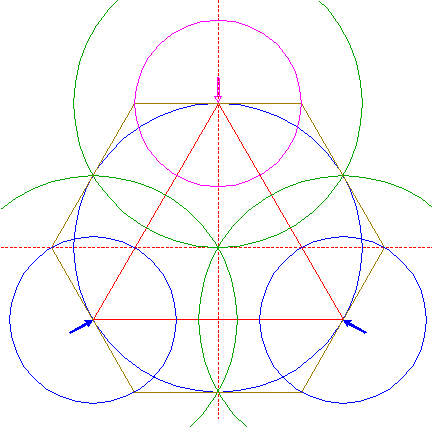Copy circle 5 two times, to the other angular points of triangle 2. 7.Construct a circle concentric to circle 5, tangent to the lower righthand circle 3 at the upper lefthand side. 8.Copy circle 7 two times, to the other angular points of triangle 2. 9.Construct a circle concentric to circle 1, passing through the intersection of circle 7 and the upper righthand side of hexagon 4. 10.Copy circle 9 three times, to the angular points of triangle 2. 11.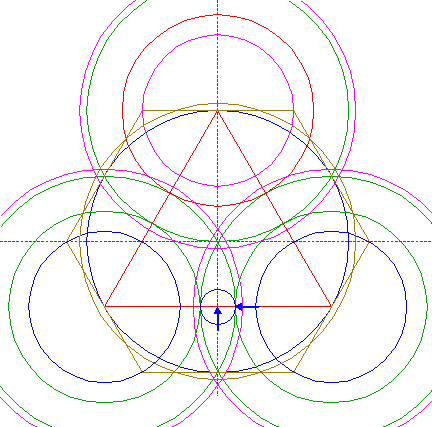Construct a circle centered at the intersection of the lower side of triangle 2 and the vertical centerline, tangent to the lower lefthand circle 3 at the righthand side. 12.Copy circle 11 to the upper intersection of circle 9 and the vertical centerline. 13.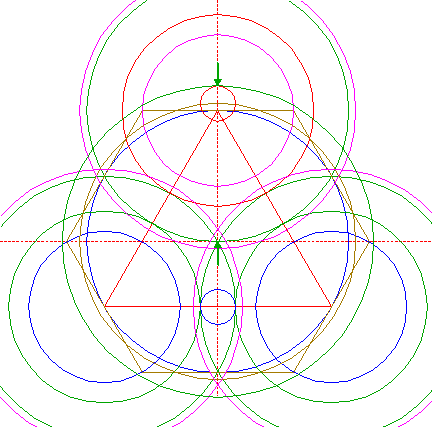Construct a circle concentric to circle 1, tangent to circle 12 at the upper side. 14.Construct a circle centered at the righthand angular point of hexagon 4, tangent to circle 13 at the righthand side. 15.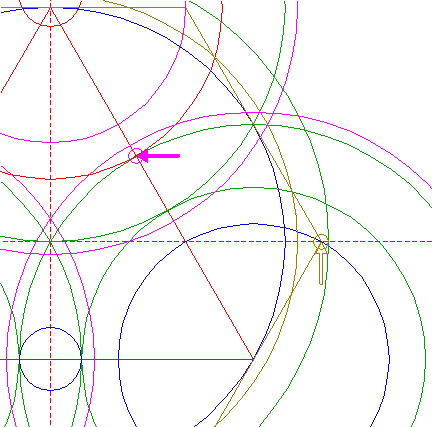Copy circle 14 to the intersection of circle 7 and the righthand side of triangle 2. 16.Construct a circle concentric to circle 5, tangent to circle 15 at the lower righthand side. 17.Copy circle 16 two times, to the other angular points of triangle 2. 18.Circles 1, 3, 5, 6, 7, 8, 9, 10, 13, 16, and 17, are used for the final reconstruction. 19.Remove all parts not visible within the formation itself. 20.Colour all areas corresponding to standing... 21.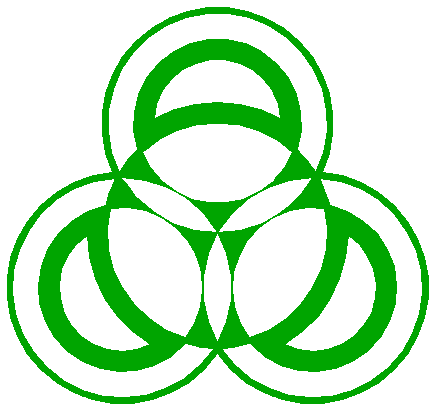...or to flattened crop, and finish the reconstruction of the 2019 Long Wood formation. 22.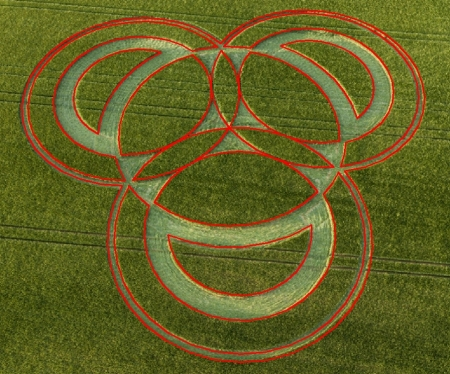The final result, matched with two aerial images.